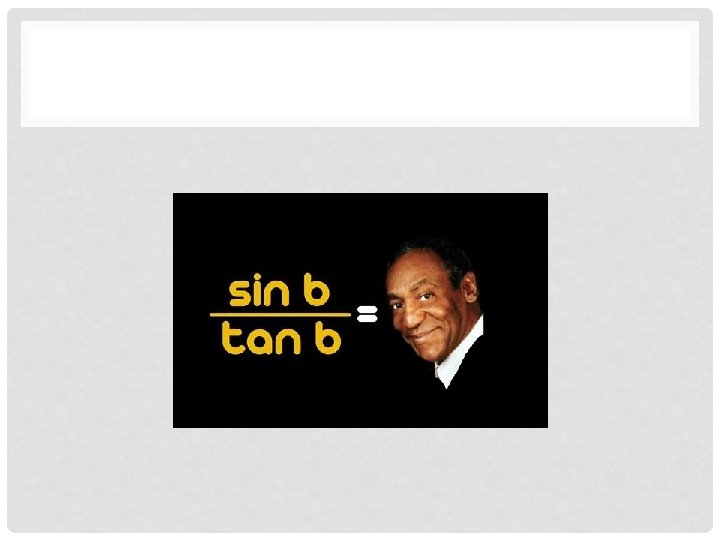# 17 BASIC TRIGONOMETRIC IDENTITIES An identity is an

• Slides: 1517. BASIC TRIGONOMETRIC IDENTITIES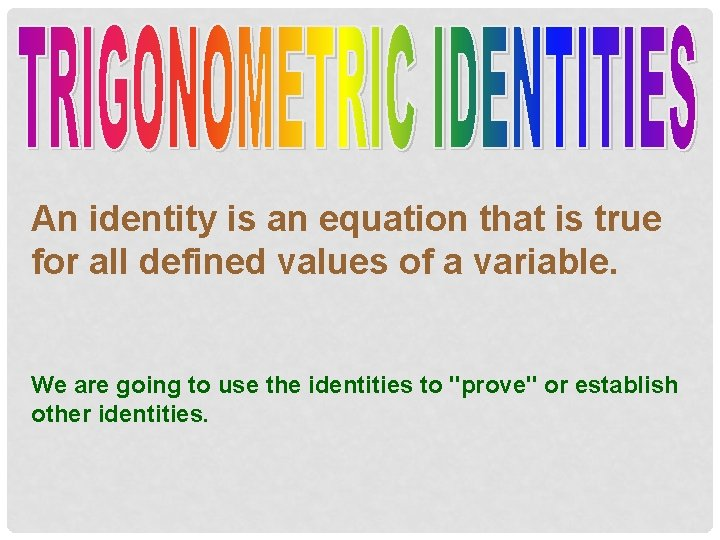An identity is an equation that is true for all defined values of a variable. We are going to use the identities to "prove" or establish other identities.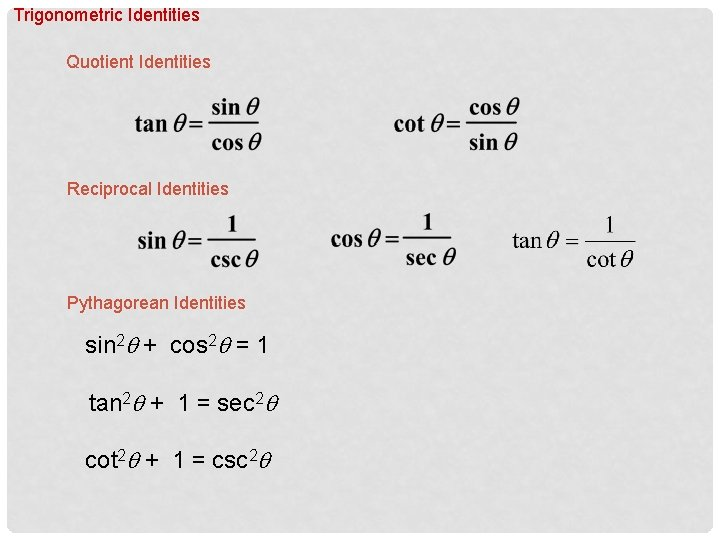Trigonometric Identities Quotient Identities Reciprocal Identities Pythagorean Identities sin 2 q + cos 2 q = 1 tan 2 q + 1 = sec 2 q cot 2 q + 1 = csc 2 q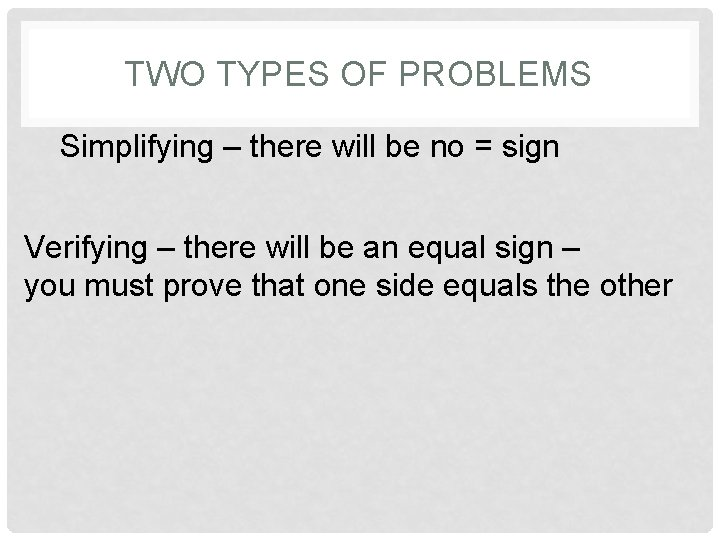TWO TYPES OF PROBLEMS Simplifying – there will be no = sign Verifying – there will be an equal sign – you must prove that one side equals the otherSIMPLIFYING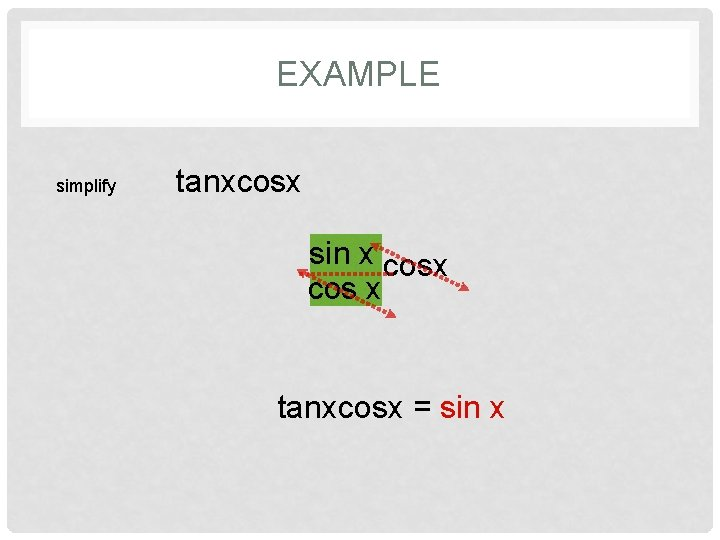EXAMPLE simplify tanxcosx sin x tanx cos x tanxcosx = sin x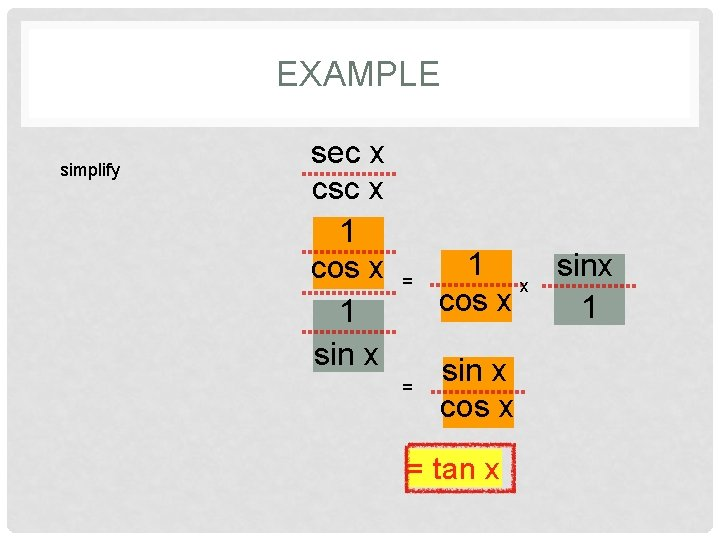EXAMPLE simplify sec x csc x 1 cos sec x csc 1 x sin x = 1 sinx x cos x 1 = sin x cos x = tan x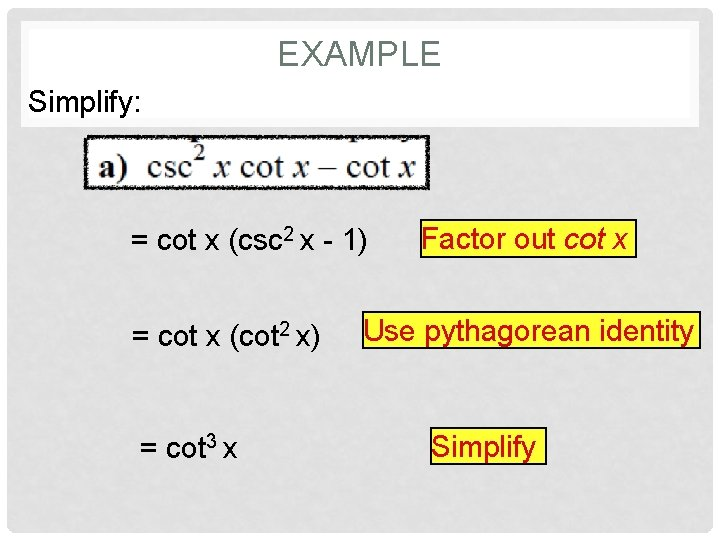EXAMPLE Simplify: = cot x (csc 2 x - 1) = cot x (cot 2 x) = cot 3 x Factor out cot x Use pythagorean identity Simplify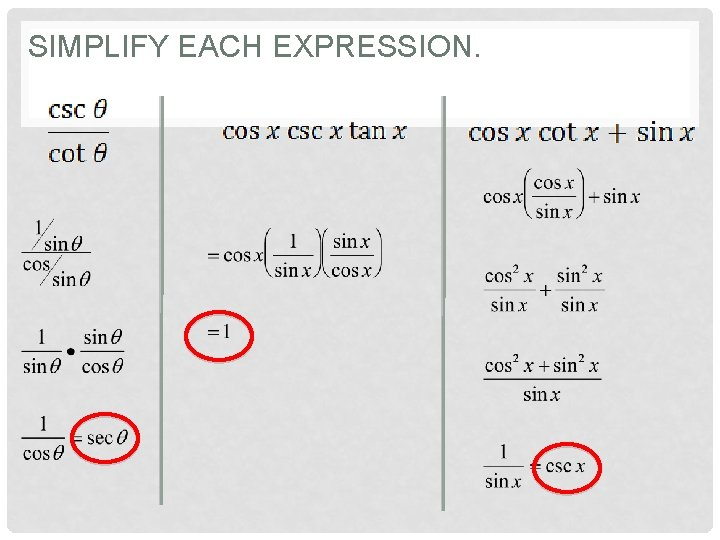SIMPLIFY EACH EXPRESSION.Simplify.Verifying In establishing an identity you should NOT move things from one side of the equal sign to the other. Instead – start with the more complicated side, substitute using identities you know, and simplifying until both sides match.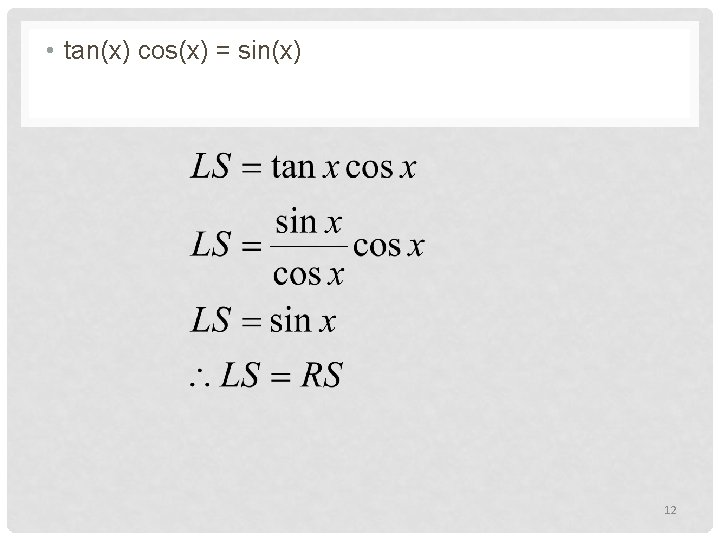• tan(x) cos(x) = sin(x) 12• tan 2(x) = sin 2(x) cos-2(x) 13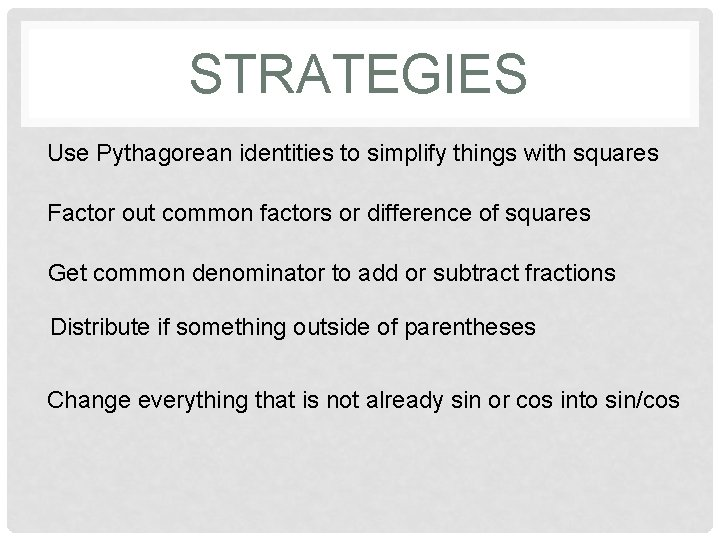STRATEGIES Use Pythagorean identities to simplify things with squares Factor out common factors or difference of squares Get common denominator to add or subtract fractions Distribute if something outside of parentheses Change everything that is not already sin or cos into sin/cos